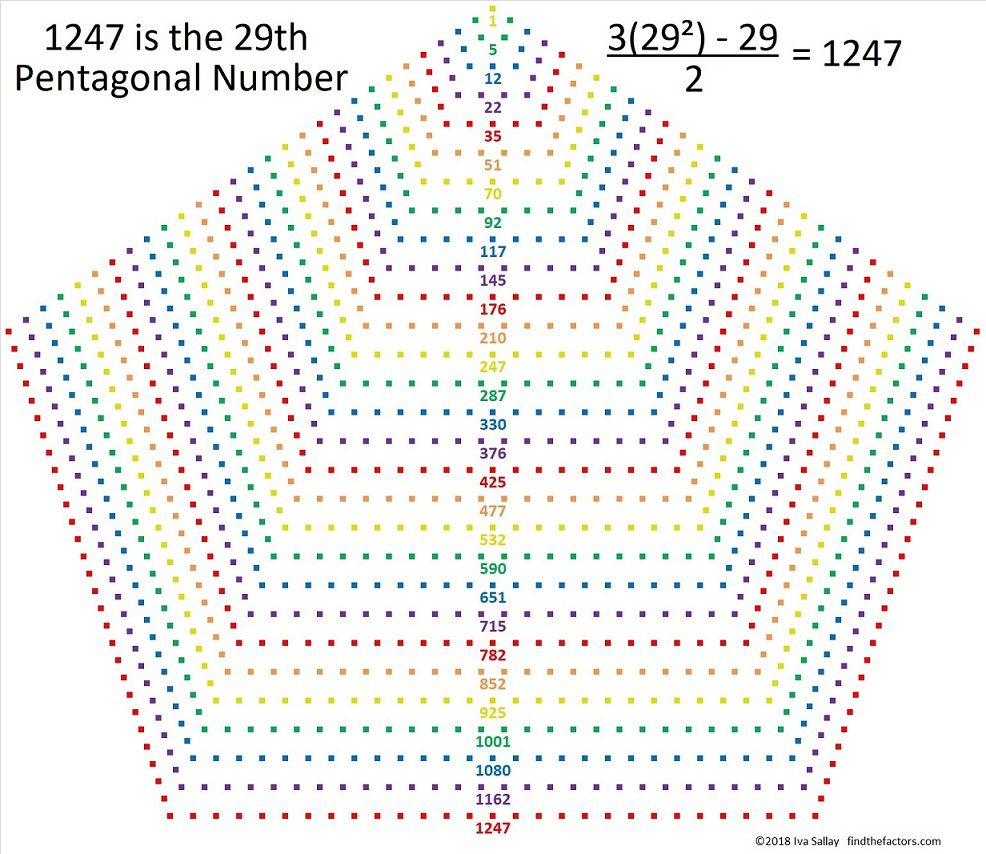# 1247 Is a Pentagonal Number

Two factors of 1247 make it the 29th pentagonal number. Here’s why:

29(3·29-1)/2 = 29(86)/2 = 29(43) = 1247

Here is an illustration of this pentagonal number featuring a different, but equivalent, formula.  Seeing the pentagonal numbers less than 1247 in the illustration won’t be difficult either.Here are some more facts about the number 1247:

• 1247 is a composite number.
• Prime factorization: 1247 = 29 × 43
• The exponents in the prime factorization are 1 and 1. Adding one to each and multiplying we get (1 + 1)(1 + 1) = 2 × 2 = 4. Therefore 1247 has exactly 4 factors.
• Factors of 1247: 1, 29, 43, 1247
• Factor pairs: 1247 = 1 × 1247 or 29 × 43
• 1247 has no square factors that allow its square root to be simplified. √1247 ≈ 35.312891247 is the sum of consecutive prime numbers two different ways:
It is the sum of the twenty-three prime numbers from 11 to 103.
It is also the sum of seven consecutive primes:
163 + 167 + 173 + 179 + 181 + 191 + 193 = 1247

1247 is the hypotenuse of a Pythagorean triple:
860-903-1247 which is (20-21-29) times 43

This site uses Akismet to reduce spam. Learn how your comment data is processed.